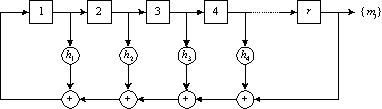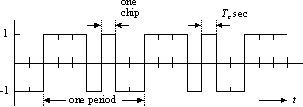### JPL's Wireless Communication Reference Website

#### Chapter: Analog and Digital Transmission Section: CDMA, Direct Sequence CDMA, Codes.# Maximum-Length Sequences

### ML code generation

To spread or despread a signal, a spreading function is used which is generated by a spreading-function generator. Here we address the generation and properties of maximal-length (ML) codes. The figure below gives an example of a r-stage shift register for the generation of ML codes.Figure: Shift register for the generation of an ML code

Such shift register implements a primitive polynomial h(x):

```h(x) = xr + h(r-1) x(r-1) + .... h1 x + 1
```
which is the generator polynomial for a code. In the polynomial h(x), the coefficient hi takes on the binary value 0 or 1. In practice the coefficients hi determine the feedback connections in the code generator. For these feedback connections tables exist. The feedback connections, or the corresponding realized polynomial, are represented by the notation
```[r, h(r-1) (r-1), ..., h1].
```
where zero-entries are not written explicitly.

###Exercise and example

Draw a [3,1] code generator which realizes the polynomial and generates [3,1] codes.

### ML code properties

The code generator outputs a binary-valued sequence {mj}. This sequence repeats itself every Nc elements. These elements are called chips. One period of the sequence, thus Nc chips, is called the pseudo noise or spreading code. In DS-CDMA practice, the sequence {mj} is transmitted mostly as a bipolar waveform p(t), called the spreading waveform. An example is given below.Figure: Waveform p(t) associated with the [3,1] code. (without modulation by a user signal)

ML codes are, by definition, the largest codes that can be generated by a shift register of a given length. For a r-stage shift register their length is Nc = 2r - 1. Thus the [3, 1] code has length Nc = 7.

An important reason for using ML codes is that they have very desirable autocorrelation properties suitable for spread-spectrum systems.Figure: Auto-correlation for an ML code with chip duration Tc and period N Tc

From the autocorrelation function for ML codes, it follows that this code is indeed suitable as spreading code for spread-spectrum systems. For the autocorrelation function has a clear maximum if t = 0, but is small for any other offset. Due to this property

• a spread-spectrum receiver can "easily" recognize code synchronization between the received code and the locally generated (identical) code by examining their correlation value, and
• a receiver locked to the dominant wave, only sees little interference from delayed waves, which may be present in a multipath channel.

### Code Properties### JPL's Wireless Communication Reference Website © Jean-Paul M.G. Linnartz1995, 1999.

 lNumber of LFSR elements m = 2l-1Sequence length Number of ml sequences Max. cross correlation,Normalized 3 7 2 0.71 4 15 2 0.60 5 31 6 0.35 6 63 6 0.36 7 127 18 0.32 8 255 16 0.37 10 1023 60 0.37 12 4095 144 0.34